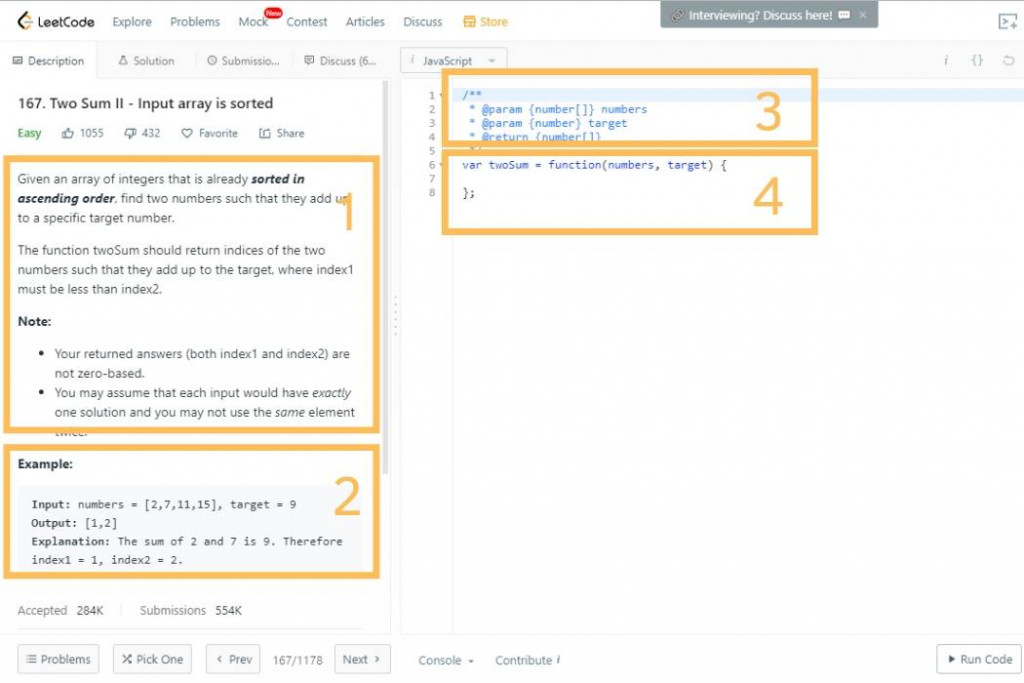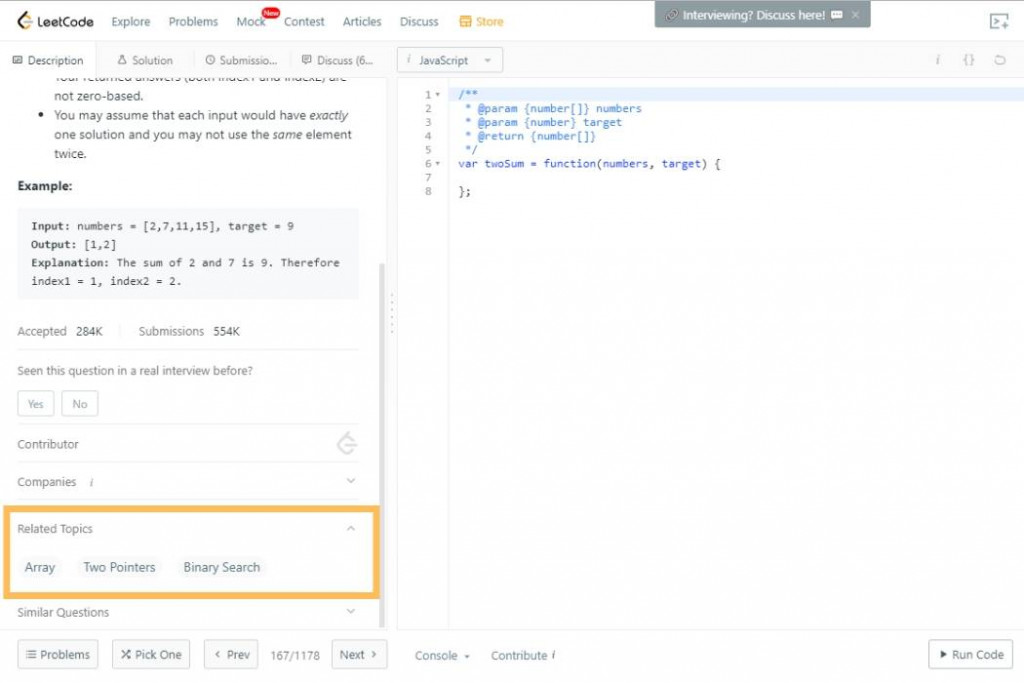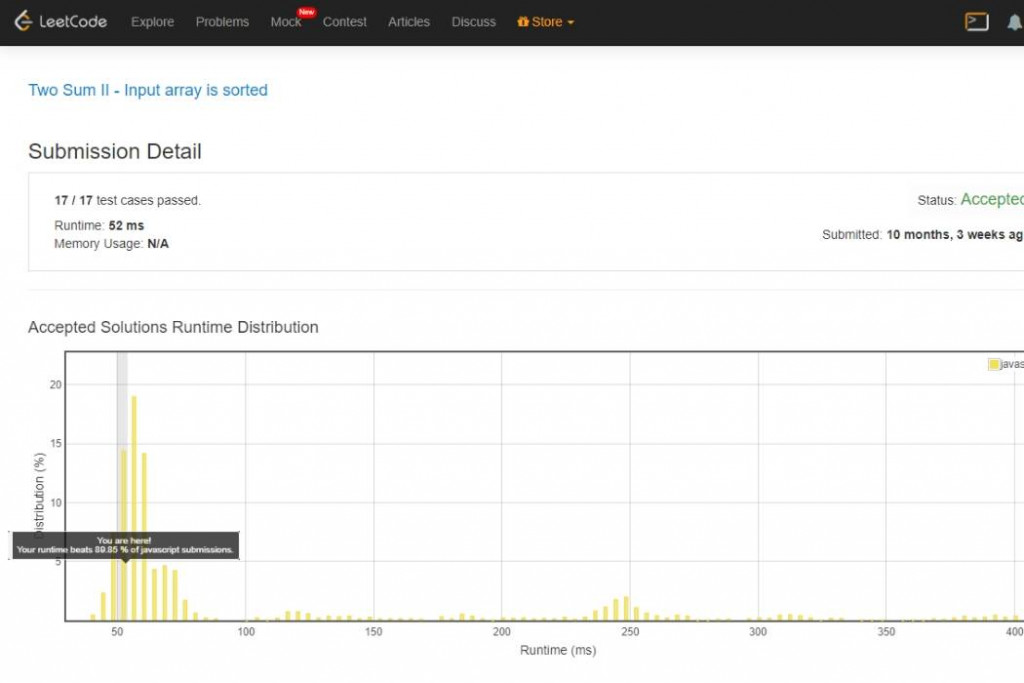DAY 5
11
Software Development

[番外篇] 解 LeetCode 之前新手刷題會遇到的困難• 台灣軟體業偏向快速完成作品，而且現在前端框架、Library、好用外掛實在太多，導致最基礎的純 JS 很不熟，但刷題沒有任何第三方工具可以使用，必須對語法本身相當熟練(例如 javaScript)。
• 現在編輯器越來越厲害，很多快速鍵。但實際面試解題沒有編輯器幫助，如果語法錯誤、打錯字並不會有提示。

怎麼開始如何刷題1&2 題目跟範例

// ------- 1. 題目 ------------
// 167. Two Sum II - Input array is sorted
// Given an array of integers that is already sorted in ascending order,
// find two numbers such that they add up to a specific target number.

// The function twoSum should return indices of the two numbers such
// that they add up to the target, where index1 must be less than index2.

// 請找已排序陣列(小到大)裡，哪兩個數字總和等於 target
// Note:

// You may assume that each input would have exactly one solution and
// you may not use the same element twice.
// Example:

// ------- 2. 範例 ------------
// Input: numbers = [2,7,11,15], target = 9
// Output: [1,2]
// Explanation: The sum of 2 and 7 is 9. Therefore index1 = 1, index2 = 2.

3 input/output

/**
* @param {number[]} numbers //[都是 number]
* @param {number} target
* @return {number[]}  // 值都是 number 的 Array
*/

解題涵式

var twoSum = function(numbers, target) {

};

Think

• 想一些"極限值"。面試時也可以問考官這些狀況會不會發生
• 稍微想一下這個題目會用甚麼方法，例如 two pointer 或 stack。Leetcode 上也有 realated topic 可以參考終於可以開始解題了

• 我會先處裡極限值，以這個範例來說，當長度 < 2 時就不處理
• 開始邊寫邊驗證各種列出來的範例
var twoSum = function(numbers, target) {
// 想極限，例如只有一個值  target = 2;  target = 9
// 很多相同的值 [2, 2, 2]
// 如果有大於兩個結果 [1, 6, 2, 5] target = 7 // 但這部分題目有說明不會發生
// 值是負的 [-1, 8] target 7 因為題目只有說是 integers

let len = numbers.length
// pointer 概念
let pointer = 0
let ind = len - 1;

if(len < 2){
return false;
}
// ... 後面省略
};

• 思考超過一小時還解不出來
• 解出來之後看看別人有沒有更好解法，然後加強自己解法結論

1. 確定問題再說什麼，想一些 edge case 問面試官會不會發生(溝通能力)
2. 想一下該題會用哪種演算法 (展現你資料結構/演算法能力)
3. 寫出 code
4. 測試
5. 不斷溝通，切記不要當一個安靜的面試者，你可以把面試官當成你平常的同事，把解題想法都先跟他討論

4 則留言

0hannahpun iT邦新手 4 級 ‧ 2019-09-06 12:06:29 檢舉

hannahpun iT邦新手 4 級 ‧ 2019-09-06 12:06:30 檢舉1

XDDDDDD

hannahpun iT邦新手 4 級 ‧ 2019-09-06 12:06:53 檢舉

0

hannahpun iT邦新手 4 級 ‧ 2019-09-07 05:11:12 檢舉1

hannahpun iT邦新手 4 級 ‧ 2020-02-23 15:12:41 檢舉